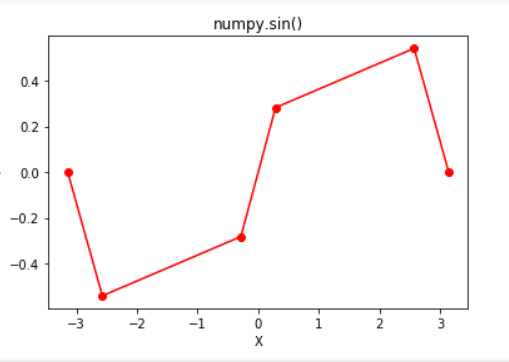# Python | math.sin() function

• Last Updated : 20 Mar, 2019

In Python, math module contains a number of mathematical operations, which can be performed with ease using the module. `math.sin()` function returns the sine of value passed as argument. The value passed in this function should be in radians.

Syntax: math.sin(x)

Attention geek! Strengthen your foundations with the Python Programming Foundation Course and learn the basics.

To begin with, your interview preparations Enhance your Data Structures concepts with the Python DS Course. And to begin with your Machine Learning Journey, join the Machine Learning - Basic Level Course

Parameter:
x : value to be passed to sin()

Returns: Returns the sine of value passed as argument

Code #1:

 `# Python code to demonstrate the working of sin()``    ` `# importing "math" for mathematical operations ``import` `math ``   ` `a ``=` `math.pi ``/` `6``    ` `# returning the value of sine of pi / 6 ``print` `(``"The value of sine of pi / 6 is : "``, end ``=``"") ``print` `(math.sin(a)) `
Output:
```The value of sine of pi/6 is : 0.49999999999999994
```

Code #2:

 `# Python program showing ``# Graphical representation of ``# sin() function ``import` `math``import` `matplotlib.pyplot as plt `` ` `in_array ``=` `[``-``3.14159265``, ``-``2.57039399``, ``-``0.28559933``,``            ``0.28559933``, ``2.57039399``,  ``3.14159265``]`` ` `out_array ``=` `[]`` ` `for` `i ``in` `range``(``len``(in_array)):``    ``out_array.append(math.sin(in_array[i]))``    ``i ``+``=` `1`` ` `  ` `print``(``"in_array : "``, in_array) ``print``(``"\nout_array : "``, out_array) `` ` `# red for numpy.sin() ``plt.plot(in_array, out_array, color ``=` `'red'``, marker ``=` `"o"``) ``plt.title(``"math.sin()"``) ``plt.xlabel(``"X"``) ``plt.ylabel(``"Y"``) ``plt.show() `
Output:

in_array : [-3.14159265, -2.57039399, -0.28559933, 0.28559933, 2.57039399, 3.14159265]

out_array : [-3.5897930298416118e-09, -0.5406408168673427, -0.2817325547837714, 0.2817325547837714, 0.5406408168673427, 3.5897930298416118e-09]My Personal Notes arrow_drop_up